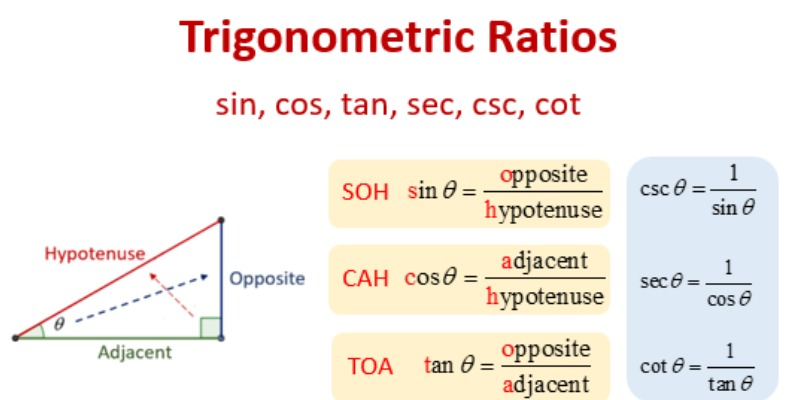### Trigonometry Trivia Quiz### Questions & Options

#### 1. Find tan P-cot R is.....

 77592 0 77593 2 77594 3 77595 1

#### 2. What is the value of tan 45?

 77596 0 77597 1 77598 4 77599 3

#### 3. The value of sin? and cos (90° – ?).....

 77600 Are same 77601 Are different 77602 No relation 77603 Information insufficient

#### 4. The value of (tan1° tan2° tan3° ... tan89°) is.....

 77604 0 77605 1 77606 2 77607 1/2

#### 5. If sin A = 1/2 and cos B = 1/2, then A + B =....

 77608 100 77609 300 77610 600 77611 900

#### 6. If cos (A + B) = 0, then sin (A – B) is reduced to......

 77612 Cos A 77613 Cos 2B 77614 Sin A 77615 Sin 2B

#### 7. If cos A = 4/5, then tan A =?

 77616 3/5 77617 4/3 77618 3/4 77619 4/5

#### 8. If sin A + sin2 A = 1, then cos2 A + cos4 A =......

 77620 1 77621 0 77622 2 77623 4

#### 9. The value of tan30°/cot60° is.....

 77624 1/v2 77625 1/v3 77626 v3 77627 1